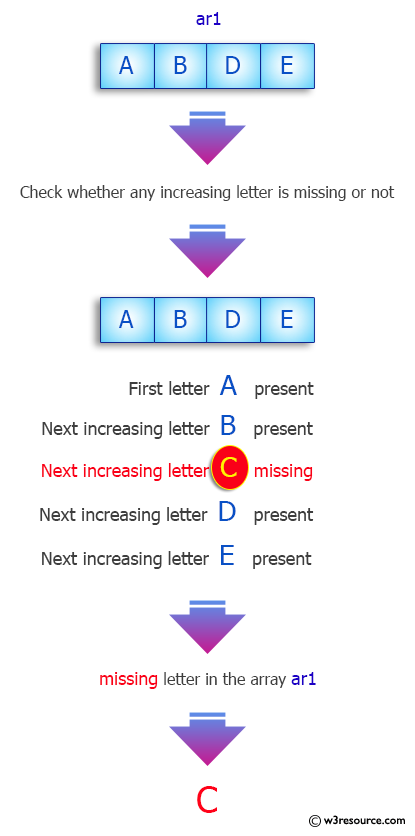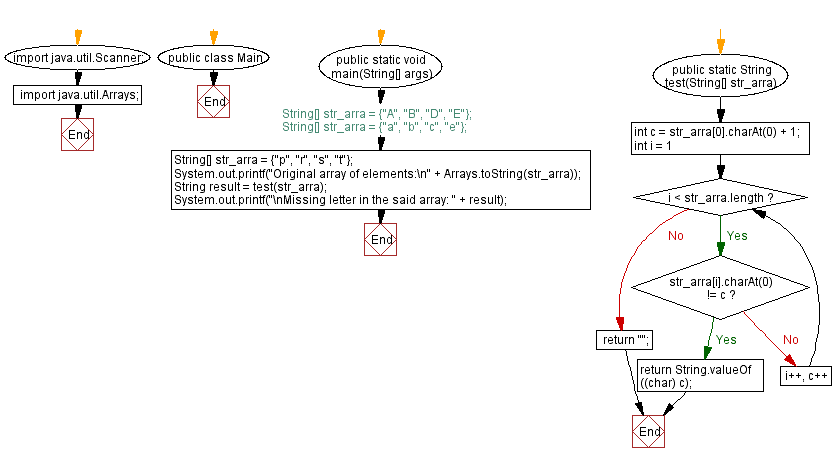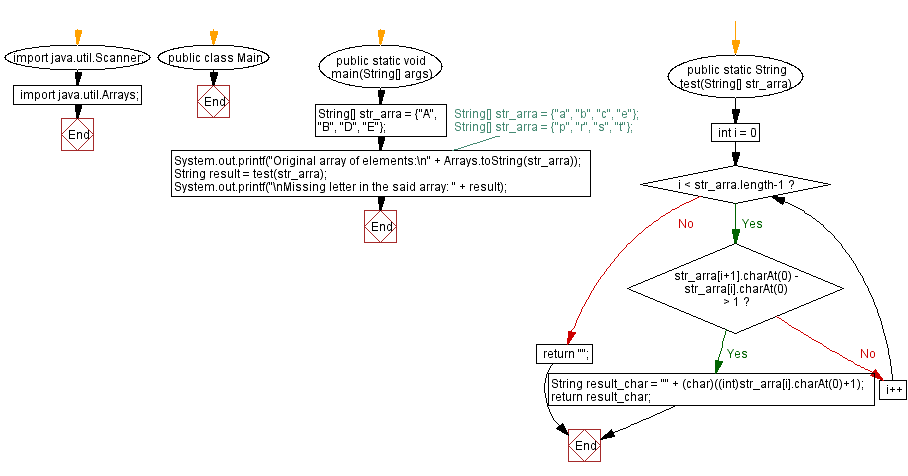﻿ Java - Missing letter from an array of increasing letters

# Java: Missing letter from an array of increasing letters

## Java Array: Exercise-79 with Solution

Write a Java program that returns the missing letter from an array of increasing letters (upper or lower). Assume there will always be one omission from the array.

Sample Data:
{"A", "B", "D", "E"} -> “C”
{"a", "b", "c", "e"} -> “d”
{"p", "r", "s", "t"} -> “q”

Pictorial Presentation:Sample Solution-1:

Java Code:

``````// Import necessary Java classes.
import java.util.Scanner;
import java.util.Arrays;

// Define the 'Main' class.
public class Main {
// Define the main method for running the program.
public static void main(String[] args) {
// Initialize an array of strings representing letters.
// You can use one of these alternative 'str_arra' arrays by uncommenting them.
// String[] str_arra = {"A", "B", "D", "E"};
// String[] str_arra = {"a", "b", "c", "e"};
String[] str_arra = {"p", "r", "s", "t"};
System.out.printf("Original array of elements:\n" + Arrays.toString(str_arra));

// Call the 'test' method to find the missing letter in the array.
String result = test(str_arra);
System.out.printf("\nMissing letter in the said array: " + result);
}

// Define the 'test' method to find the missing letter in the array.
public static String test(String[] str_arra) {
// Calculate the expected character code for the missing letter.
int c = str_arra.charAt(0) + 1;

// Iterate through the array and check for the missing letter.
for (int i = 1; i < str_arra.length; i++, c++) {
if (str_arra[i].charAt(0) != c) {
return String.valueOf((char) c);
}
}

// Return an empty string if no letter is missing.
return "";
}
}
```
```

Sample Output:

```Original array of elements:
[p, r, s, t]
Missing letter in the said array: q
```

Flowchart:Sample Solution-2:

Java Code:

``````// Import necessary Java classes.
import java.util.Scanner;
import java.util.Arrays;

// Define the 'Main' class.
public class Main {
// Define the main method for running the program.
public static void main(String[] args) {
// Initialize an array of strings representing letters.
// You can use one of these alternative 'str_arra' arrays by uncommenting them.
String[] str_arra = {"A", "B", "D", "E"};
// String[] str_arra = {"a", "b", "c", "e"};
// String[] str_arra = {"p", "r", "s", "t"};

// Print the original array of elements.
System.out.printf("Original array of elements:\n" + Arrays.toString(str_arra));

// Call the 'test' method to find the missing letter in the array.
String result = test(str_arra);

// Print the missing letter found in the array.
System.out.printf("\nMissing letter in the said array: " + result);
}

// Define the 'test' method to find the missing letter in the array.
public static String test(String[] str_arra) {
// Iterate through the array to find the missing letter.
for (int i = 0; i < str_arra.length - 1; i++) {
// Check the difference between adjacent characters.
if (str_arra[i + 1].charAt(0) - str_arra[i].charAt(0) > 1) {
// Calculate and return the missing character as a string.
String result_char = "" + (char)((int)str_arra[i].charAt(0) + 1);
return result_char;
}
}

// Return an empty string if no letter is missing.
return "";
}
}
```
```

Sample Output:

```Original array of elements:
[A, B, D, E]
Missing letter in the said array: C
```

Flowchart:Java Code Editor:

Previous Java Exercise: Negative Dominant.
Next Java Exercise: Java String Exercises

What is the difficulty level of this exercise?

Test your Programming skills with w3resource's quiz.

﻿